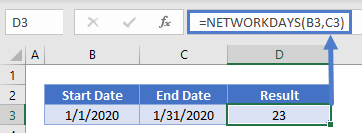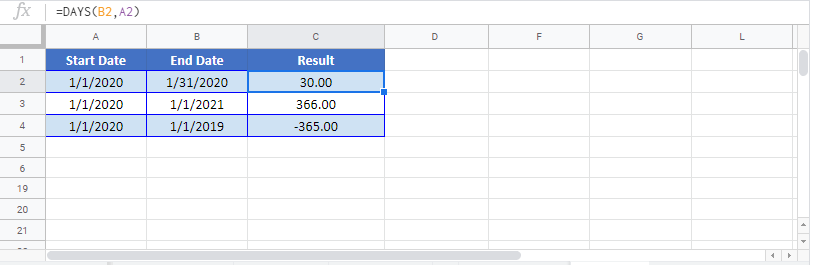# DAYS Function – Count Days in Excel, VBA, Google Sheets

This tutorial demonstrates how to use the Excel DAYS Function in Excel to count the number of days between dates.

## DAYS Function Overview

The DAYS Function Returns the number of days between two dates.

To use the DAYS Excel Worksheet Function, select a cell and type: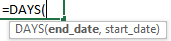(Notice how the formula inputs appear)

## Syntax and Arguments:

end_date – The end date in Excel serial number format or entered as a date with quotations (“s) surround the date. Example: You can not enter 11/12/2015 directly into the cell. Instead you need to enter “11/12/2015” or you would need to use the corresponding serial number: 42320. Alternatively, you can reference a cell with the date 11/12/2015 entered. Excel automatically converts dates stored in cells into serial format (unless the date is entered as text).

start_date – The start date in Excel serial number format or entered as a date with quotations (“s) surround the date. Example: You can not enter 11/12/2015 directly into the cell. Instead you need to enter “11/12/2015” or you would need to use the corresponding serial number: 42320. Alternatively, you can reference a cell with the date 11/12/2015 entered. Excel automatically converts dates stored in cells into serial format (unless the date is entered as text).

## Calculate Number of Days Between Dates

The DAYS Function returns the number of days between two dates. The DAYS Function even works with dates stored as text. It returns a positive number if the end_date is after the start_date: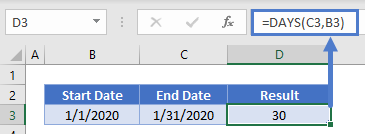or a negative number of the end_date is before the start_date:### Absolute Date Difference

To calculate the absolute difference between two dates (no negative values) surround the DAYS Function with the ABS Function:

###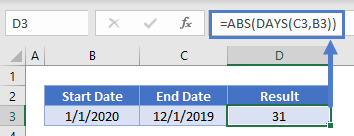Subtract Dates

Instead of using the DAYS Function, you can usually simply subtract two dates to calculate the number of days between them: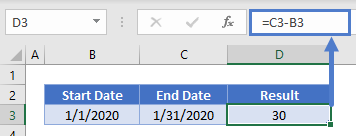However, this won’t work with dates stored as text.

Also, this method might return an unexpected result if a time value is stored with the dates. Read our article on calculating the difference between dates to learn more.

### Days From Today

To calculate the number of days between a date and Today use the TODAY Function as one of the dates:

###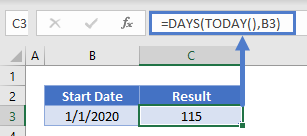DAYS360 Function

Instead if you need to calculate the number of days between dates using a 360 day year (where each month has 30 days), use the DAYS360 Function: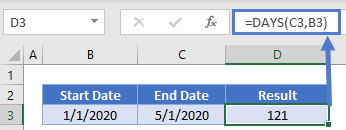###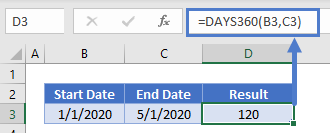NETWORKINGDAYS Function

The NETWORKINGDAYS Function will calculate the number of business days between dates: